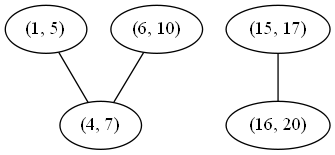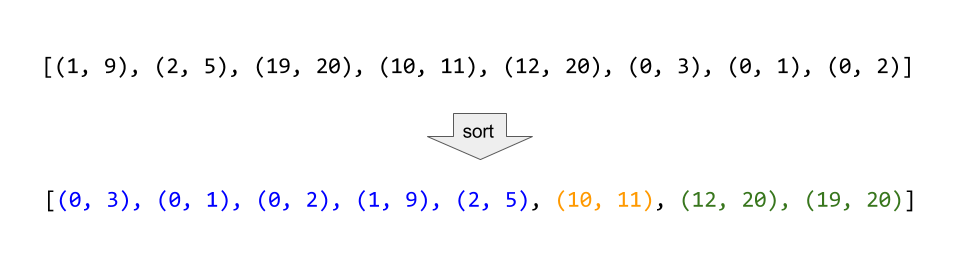## Solution

#### Approach 1: Connected Components

Intuition

If we draw a graph (with intervals as nodes) that contains undirected edges between all pairs of intervals that overlap, then all intervals in each connected component of the graph can be merged into a single interval.

Algorithm

With the above intuition in mind, we can represent the graph as an adjacency list, inserting directed edges in both directions to simulate undirected edges. Then, to determine which connected component each node is it, we perform graph traversals from arbitrary unvisited nodes until all nodes have been visited. To do this efficiently, we store visited nodes in a Set, allowing for constant time containment checks and insertion. Finally, we consider each connected component, merging all of its intervals by constructing a new Interval with start equal to the minimum start among them and end equal to the maximum end.

This algorithm is correct simply because it is basically the brute force solution. We compare every interval to every other interval, so we know exactly which intervals overlap. The reason for the connected component search is that two intervals may not directly overlap, but might overlap indirectly via a third interval. See the example below to see this more clearly.Although (1, 5) and (6, 10) do not directly overlap, either would overlap with the other if first merged with (4, 7). There are two connected components, so if we merge their nodes, we expect to get the following two merged intervals:

(1, 10), (15, 20)

Complexity Analysis

• Time complexity :

Building the graph costs time, as in the worst case all intervals are mutually overlapping. Traversing the graph has the same cost (although it might appear higher at first) because our visited set guarantees that each node will be visited exactly once. Finally, because each node is part of exactly one component, the merge step costs time. This all adds up as follows:

• Space complexity :

As previously mentioned, in the worst case, all intervals are mutually overlapping, so there will be an edge for every pair of intervals. Therefore, the memory footprint is quadratic in the input size.

#### Approach 2: Sorting

Intuition

If we sort the intervals by their start value, then each set of intervals that can be merged will appear as a contiguous "run" in the sorted list.

Algorithm

First, we sort the list as described. Then, we insert the first interval into our merged list and continue considering each interval in turn as follows: If the current interval begins after the previous interval ends, then they do not overlap and we can append the current interval to merged. Otherwise, they do overlap, and we merge them by updating the end of the previous interval if it is less than the end of the current interval.

A simple proof by contradiction shows that this algorithm always produces the correct answer. First, suppose that the algorithm at some point fails to merge two intervals that should be merged. This would imply that there exists some triple of indices , , and in a list of intervals such that and (, ) can be merged, but neither (, ) nor (, ) can be merged. From this scenario follow several inequalities:

We can chain these inequalities (along with the following inequality, implied by the well-formedness of the intervals: ) to demonstrate a contradiction:

Therefore, all mergeable intervals must occur in a contiguous run of the sorted list.Consider the example above, where the intervals are sorted, and then all mergeable intervals form contiguous blocks.

Complexity Analysis

• Time complexity :

Other than the sort invocation, we do a simple linear scan of the list, so the runtime is dominated by the complexity of sorting.

• Space complexity : (or )

If we can sort intervals in place, we do not need more than constant additional space. Otherwise, we must allocate linear space to store a copy of intervals and sort that.